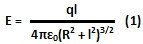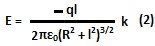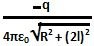## Wednesday, November 17, 2010

### Irodov Problem 3.62Using the method of images we can replace the conductor by an image of the positively charged ring i.e. a negatively charged ring at a distance 2l from the original ring as shown in the figure.

From problem 3.9, we know the electric field due to a positively charged ring at a distance l along the axis as,The net electric field due to both the rings (image and real) on the conductor at the center of the two rings is given by,As seen from problem 3.59, if the surface charge density at the conductor isat this location then we have,b)The electric field strength at the center of the ring will be entirel due to negatively charged image ring and can be computed using eqn (1) as,The electric potential due the positively charged ring at its center is given by,. The electric potential due to the negatively charged ring at the center of the positively charged ring is given by,. The net potential is thus given by,